# 数据结构 - 栈的基本操作+四则运算（后缀表达式）

7 篇文章 0 订阅

10月21日晨刷完之后更新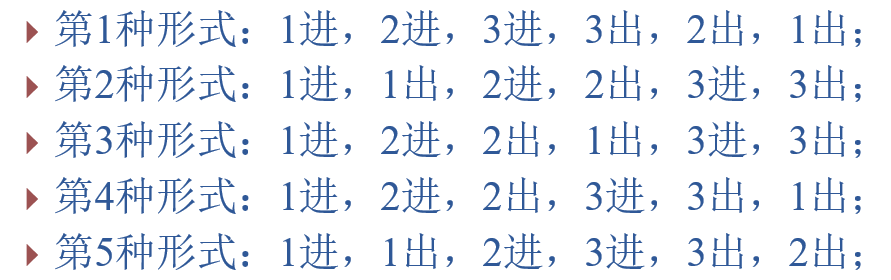top=0表示栈内只有一个元素，top=-1表示空栈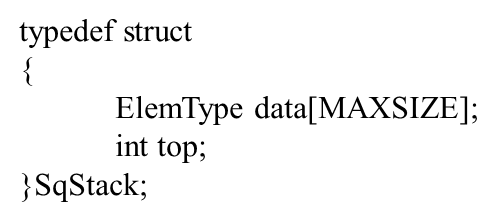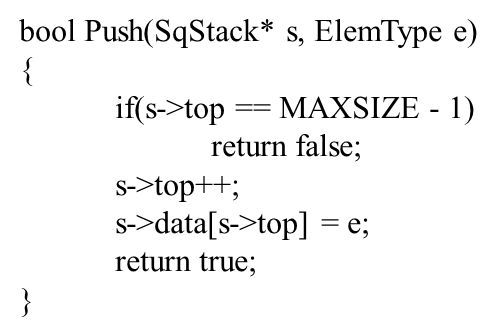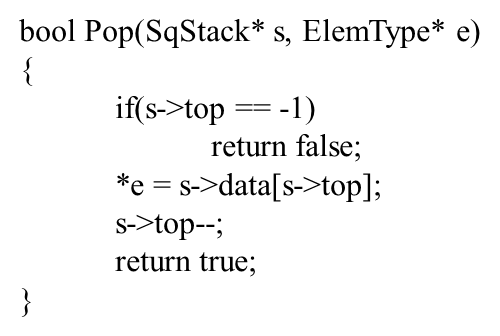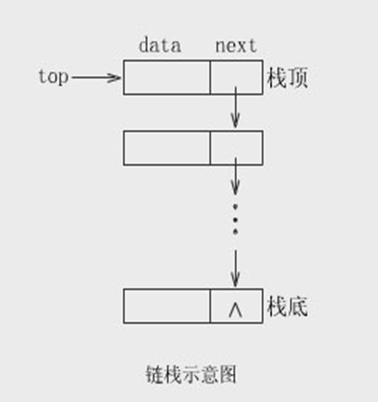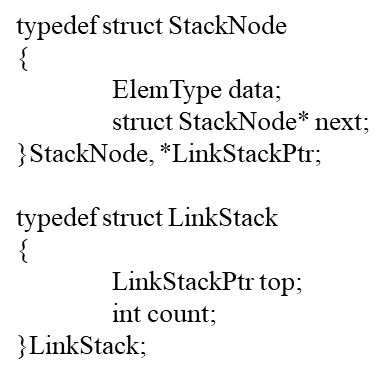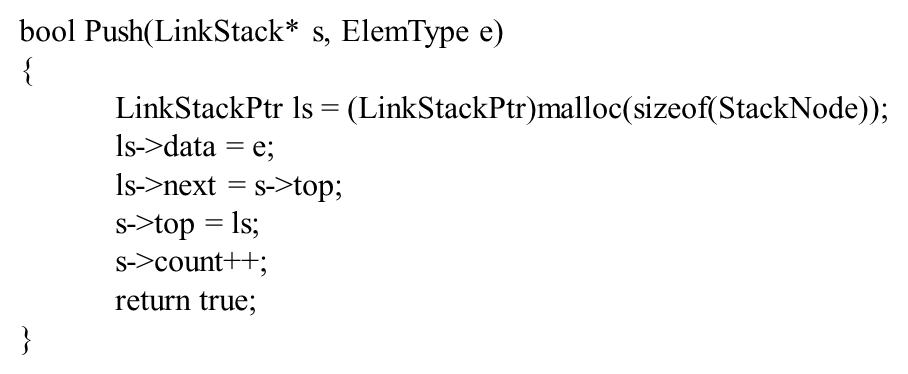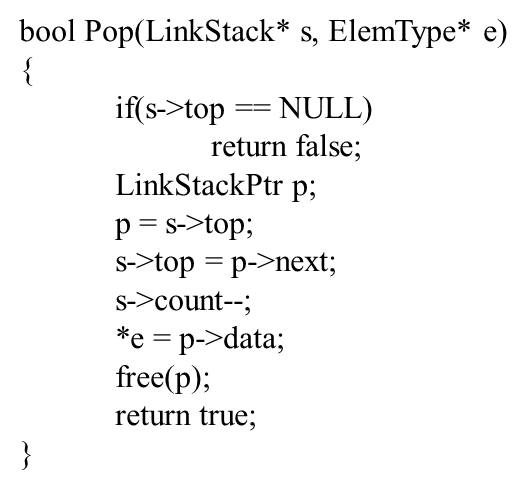（问：有代码吗

#include <stdio.h>
#include <stdlib.h>

#define OK     1
#define ERROR  -1
struct node
{
int data;
struct node *next;
};
typedef struct node Node;

struct stack
{
Node *top;
int count;
};
typedef struct stack Stack;

int InitStack(Stack *l)
{
l->top = NULL;
l->count = 0;

return OK;
}

int EmptyStack(Stack *s)
{
return (s->count == 0) ? OK : ERROR;
}

int Push(Stack *s, int e)
{
//……
}

int GetTop(Stack *s)
{
//……
}

int Priority(char s)
{
switch(s)
{
case '(':
return 3;
case '*':
case '/':
return 2;
case '+':
case '-':
return 1;
default:
return 0;
}
}

int Pop(Stack *s)
{
//……
}

int main()
{
Stack num, opt;
char str={0};
int i = 0, tmp = 0, j;
if(InitStack(&num) != OK || InitStack(&opt) != OK)
{
printf("Init Failure!\n");
return 0;
}
scanf("%s",str);
while(str[i] != '\0' || EmptyStack(&opt) != OK)
{
if(str[i] >= '0' && str[i] <= '9')
{
tmp = tmp * 10 + str[i] - '0';
i++;
if(str[i] < '0' || str[i] > '9')
{
Push(&num,tmp);
tmp = 0;
}
}
else
{
if((EmptyStack(&opt) == OK) || (GetTop(&opt) == '(' && str[i] != ')') || Priority(str[i]) > Priority(GetTop(&opt)))//进栈不参与运算
{
Push(&opt,str[i]);
i++;
continue;
}
if(GetTop(&opt) == '(' && str[i] == ')')
{
Pop(&opt);
i++;
continue;
}
if((str[i] == '\0' && EmptyStack(&opt) != OK) || (str[i] == ')' && GetTop(&opt) != '(') || Priority(str[i]) <= Priority(GetTop(&opt)))//出栈并参与运算
{
switch(Pop(&opt))
{
case '+':
Push(&num, Pop(&num) + Pop(&num));
break;
case '-':
j = Pop(&num);
Push(&num, Pop(&num) - j);
break;
case '*':
Push(&num, Pop(&num) * Pop(&num));
break;
case '/':
j = Pop(&num);
Push(&num, Pop(&num) / j);
}
continue;
}
}
}
printf("%d\n",Pop(&num));
return 0;
}

07-07
05-1501-171445
06-03
09-261976
06-073302
02-251176
12-231万+
11-04243
04-09112
09-0194
02-05314
12-101万+
05-06
07-07
07-3022
09-101544
04-23134点击重新获取扫码支付余额充值